## Trigonometry and Complex Exponentials

Amazingly, trig functions can also be expressed back in terms of the complex exponential. Then everything involving trig functions can be transformed into something involving the exponential function. This is very surprising.

In order to easily obtain trig identities like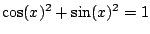, let's write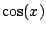and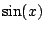as complex exponentials. From the definitions we have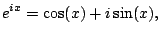so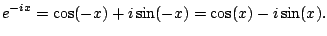Adding these two equations and dividing by 2 yields a formula for, and subtracting and dividing by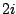gives a formula for: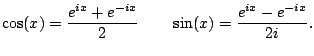We can now derive trig identities. For example,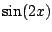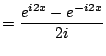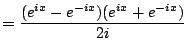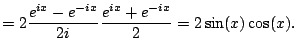I'm unimpressed, given that you can get this much more directly using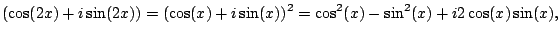and equating imaginary parts. But there are more interesting examples.

Next we verify that (4.4.1) implies that. We have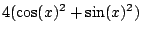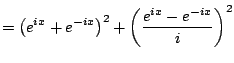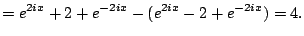The equality just appears as a follow-your-nose algebraic calculation.

Example 4.4.4   Compute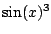as a sum of sines and cosines with no powers.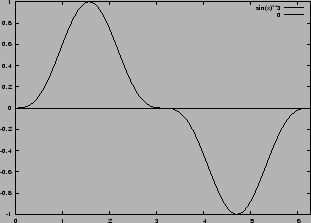Solution. We use (4.4.1):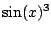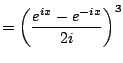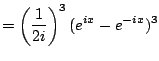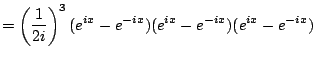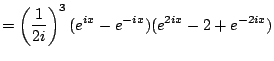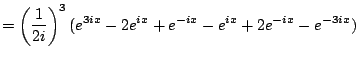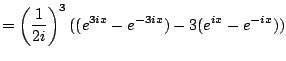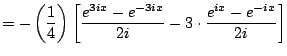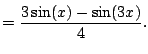William Stein 2006-03-15